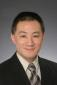# Rti dds conversion from C++ to matlab

2 posts / 0 new
Offline
Last seen: 2 years 2 months ago
Joined: 05/20/2020
Posts: 1
Rti dds conversion from C++ to matlab

Hello Everyone,

Class class1;
std::vector<class2>array{x,y,z};
class1.array2(rti::core::vector<class2>(array));

what does the last line mean and how can I change this c++ line to Matlab?

Keywords:Offline
Last seen: 15 hours 34 min ago
Joined: 11/29/2012
Posts: 399

Sorry to just have seen this post.  Where the the code that you posted come from?  What does it mean to convert C++ to Matlab?

If the code that you posted is supposed to be C++, then

class1.array2() is some method in class1 that takes an argument.

In the code, that argument is "rti::core::vector<class2>(array)", which looks like it's a constructor of a vector class whose elements are of type "class2" using the argument "array" in the construction.  And then "array" is a "std::vector<class2>", which is a vector of class 2 objects.

Have no idea why someone would write this code in C++, neverless want to translate this into Matlab...